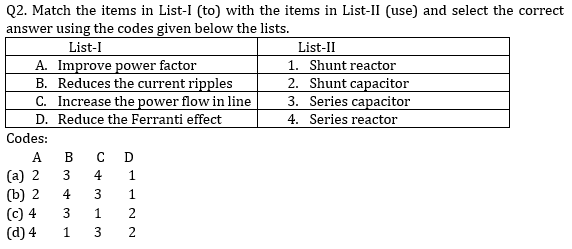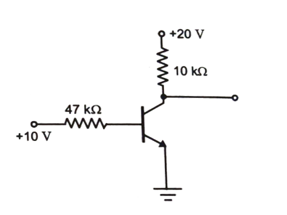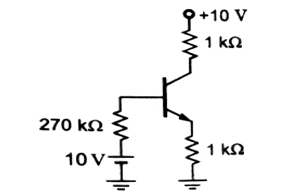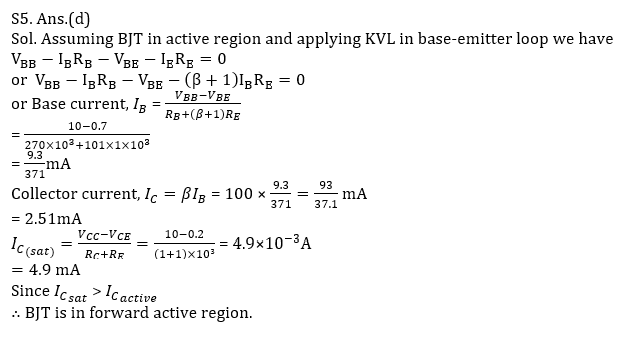Engineering Jobs   »   ELECTRICAL GATE QUIZ

# GATE’22 EE:- Daily Practice Quiz 06-Aug-2021

Each question carries 2 marks
Negative marking: 0.66 mark

Total Questions: 05
Total marks: 10
Time: 12 min.

Q1. In an electrodynamometer type wattmeter
1. Control torque is provided by spring
2. Fixed coil is iron-cored
3. Moving coil is air-cored
4. Eddy current damping is used
5. The pressure coil should be highly inductive
Which of the above statements are true?
(a) 1,2,3,4 and 5
(b) 2,3,4 and 5
(c) 1 and 3 only
(d) 1, 3 and 4 onlyQ3. An ideal transformer has a 150-turn primary and 750 turn secondary. The primary is connected to a 240 V, 50 Hz source. The secondary winding supplies a load of 4 A at a lagging power factor of 0.8. its current in the primary is
(a) 2 A
(b) 20 A
(c) 10 A
(d) 30 A

Q4. In the transistor circuit shown below, the collector-to-ground voltage is +20 V. The possible condition is(a) collector-emitter terminal shorted.
(b) emitter-to-ground connection open.
(c) 10 k Ω resistor open.
(d) collector-base terminals shorted.

Q5. The common emitter forward current gain of the transistor shown is β_F = 100.The transistor is operation in
(a) Saturation region.
(b) Cutoff region.
(c) Reverse active region.
(d) Forward active region.

SOLUTIONS
S1. Ans.(c)
Sol. In an electro-dynamometer type wattmeter:
Control torque is provided by spring
Air friction damping is used
Both fixed coil and moving coil is air-cored
Pressure coil is non-inductive
Fixed coil/current coil is connected in series
moving/pressure/potential coil is connected across load.

S2. Ans.(b)
Sol.
Shunt reactors are connected at receiving end to improve voltage profile.
Shunt capacitors are connected at the load end to improve pf of the system.
Series reactors are connected in series with line and reduces current ripples.
Series capacitor increases power flow in the line by reducing the net transfer reactance.

S3. Ans.(b)
Sol. I_1/I_2 =N_2/N_1 ⇒I_1=4×750/150=20 A

S4. Ans.(b)
Sol. Given collector to ground voltage
= 20 V = V_CC
This is possible only if I_C=I_E = 0
or emitter-to-ground connection open.Sharing is caring!